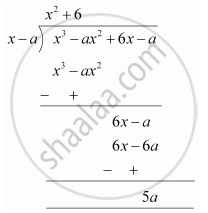# Find the remainder when x^3 – ax^2 + 6x – a is divided by x – a. - Mathematics

Find the remainder when x3 – ax2 + 6x – a is divided by x – a.

#### Solution 1

Let p(x) = x3 – ax2 + 6x – a

x - a = 0

∴ x = a

∴ Remainder = (a)3 - a(a)2 + 6(a) - a

= a3 - a3 + 6a - a

= 5a

Therefore, the remainder obtained is 5a.

#### Solution 2

By long division,Therefore, when x3 − ax2 + 6x − is divided by x − a, the remainder obtained is 5a.

Concept: Remainder Theorem
Is there an error in this question or solution?

#### APPEARS IN

NCERT Class 9 Maths
Chapter 2 Polynomials
Exercise 2.3 | Q 2 | Page 40